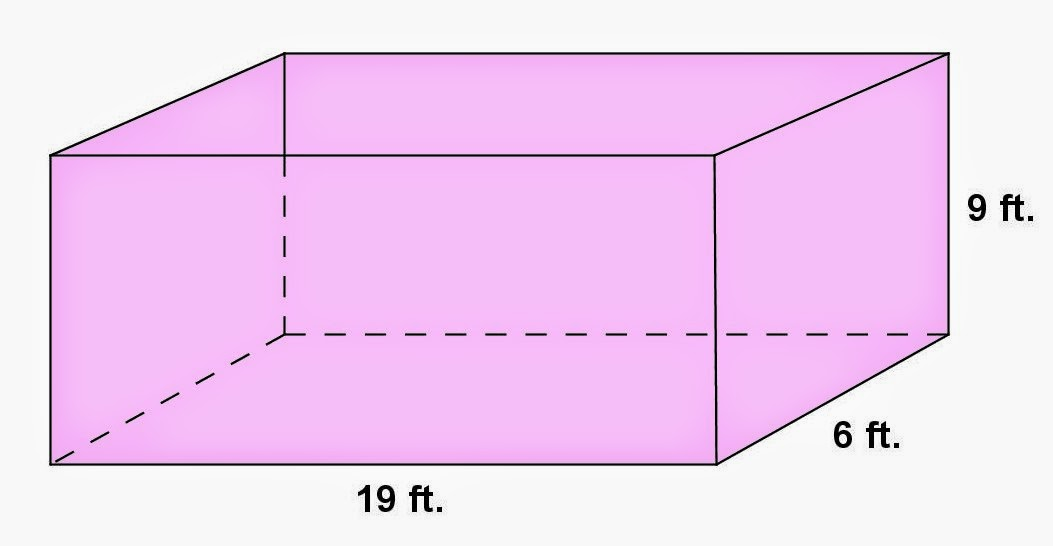## Saturday, June 7, 2014

### Rectangular Parallelepiped Problem, 3

Category: Solid Geometry, Physics

"Published in Vacaville, California, USA"

Counting 38 cu. ft. of coal to a ton, how many tons will a coal bin 19 ft. long, 6 ft. wide, and 9 ft. deep contain, when level full?

Solution:

To illustrate the problem, it is better to draw the figure as followsPhoto by Math Principles in Everyday Life

The volume of a rectangular parallelepiped is given by the formula

Substitute the values of length, width, and height of a bin, we have

The density of a substance is given by the formula

where ρ is the density, W is the weight, and V is the volume of a substance respectively.

Therefore, the weight of a coal in a bin is